# Sum of GP members

Determine the sum of the GP 30, 6, 1.2, to 5 terms. What is the sum of all terms (to infinity)?

s5 =  37.488
s =  37.5

### Step-by-step explanation: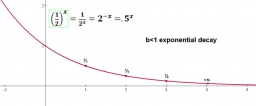Did you find an error or inaccuracy? Feel free to write us. Thank you!## Related math problems and questions:

• Two geometric progressions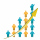Insert several numbers between numbers 6 and 384 so that they form with the given GP numbers and that the following applies: a) the sum of all numbers is 510 And for another GP to apply: b) the sum of entered numbers is -132 (These are two different geome
• GP membersThe geometric sequence has 10 members. The last two members are 2 and -1. Which member is -1/16?
• GP - three membersThe second and third of a geometric progression are 24 and 12(c+1), respectively, given that the sum of the first three terms of progression is 76. determine the value of c.
• Sum of seriesDetermine the 6-th member and the sum of a geometric series: 5-4/1+16/5-64/25+256/125-1024/625+....
• Geometric progressiobIf the sum of four consective terms of geometric progression is 80 and arithmetic mean of second and fourth term is 30 then find terms?
• Sequences AP + GPThe three numbers that make up the arithmetic sequence have the sum of 30. If we subtract from the first 5, from the second 4 and keep the third, we get the geometric sequence. Find AP and GP members.
• Geometric progressionIn geometric progression, a1 = 7, q = 5. Find the condition for n to sum first n members is sn≤217.
• Five element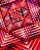The geometric sequence is given by quotient q = 1/2 and the sum of the first six members S6 = 63. Find the fifth element a5.
• InfinityIn a square with side 19 is inscribed circle, the circle is inscribed next square, again circle, and so on to infinity. Calculate the sum of the area of all these squares.
• What are 2What are the two more terms of the GP a, ax, ax2, ax3, __, __?
• Three members GPThe sum of three numbers in GP (geometric progression) is 21 and the sum of their squares is 189. Find the numbers.
• Sum 1-6Find the sum of the geometric progression 3, 15, 75,… to six terms.
• GP - sequence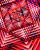The first three terms of a geometric sequence are as follows 10, 30. 90, find the next two terms of this sequence.
• Population growth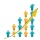How many people will be on Earth from two people for 5,000 years, if every couple has always 4 children, (2 boys and 2 girls) at the age of 25-35, and every man will live 75 years?
• Terms of GPWhat is the 6th term of the GP 9, 81, 729,. .. ?
• Infinite decimalImagine the infinite decimal number 0.99999999 .. ... ... ... That is a decimal and her endless series of nines. Determine how much this number is less than the number 1. Thank you in advance.
• What is 10What is the 5th term, if the 8th term is 80 and common ratio r =1/2?